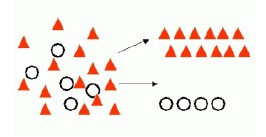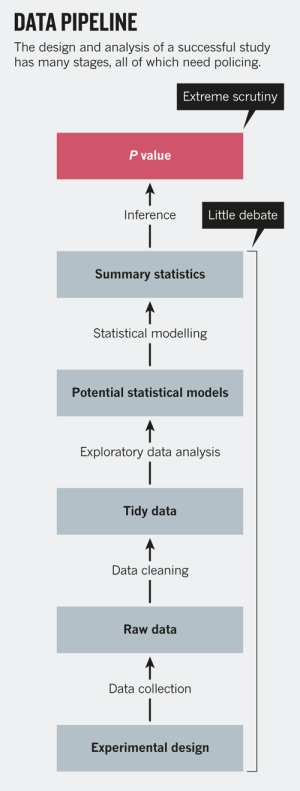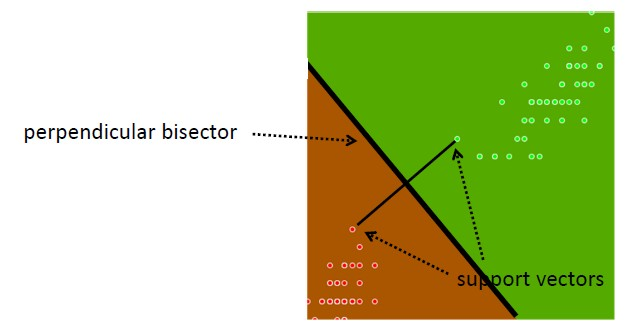# Machine Learning - (Supervised|Directed) Learning ( Training ) (Problem)

Supervised Learning has the goal of predicting a value (outcome) from particular characteristics (predictors) that describes some behaviour.

The attribute used to trained and being predicted is called the Target (outcome, …) attribute.

When the outcome attribute is:

Supervised learning is also known as directed learning. Directed data mining attempts to explain the behaviour of the target as a function of a set of independent attributes or predictors.

Supervised learning generally results in predictive models. This is in contrast to unsupervised learning where the goal is pattern detection.

The building of a supervised model involves training with a training data set of observations.

In supervised learning, you infer the general equation. For instance in linear regression, you infer the m and b of the equation y = bx+ m from the x and y

## Objectives

Recommended Pages(Machine|Statistical) Learning - (Predictor|Feature|Regressor|Characteristic) - (Independent|Explanatory) Variable (X)

A Independent variable is a variable used in supervised analysis in order to predict an outcome variable. It's also known as: Predictor Input variable, Regressors, Explanatory variable, CovariateCovariates...(Machine|Statistical) Learning - (Target|Learned|Outcome|Dependent|Response) (Attribute|Variable) (Y|DV)

An (outcome|dependent) variable is ameasure that we want to predict. : the original score collected : the predicted score (or estimator) from the equation. The hat means “estimated” from the...(Statistics|Probability|Machine Learning|Data Mining|Data and Knowledge Discovery|Pattern Recognition|Data Science|Data Analysis)

The terms pattern recognition, machine learning, data mining and knowledge discovery in databases (KDD) are hard to separate, as they largely overlap in their scope.Machine Learninsupervised learning methodKDD...Data Mining - (Classifier|Classification Function)

A classifier is a Supervised function (machine learning tool) where the learned (target) attribute is categorical (“nominal”) in order to classify. It is used after the learning process to classify...Data Mining - (Function|Model)

The model is the function, equation, algorithm that predicts an outcome value from one of several predictors. During the training process, the models are build. A model uses a logic and one of several...Data Mining - (Life cycle|Project|Data Pipeline)

Data mining is an experimental science. Data mining reveals correlation, not causation. With good data, you will make good algorithm. The most preferable solution is then to work on good features....Data Mining - Intrusion detection systems (IDS) / Intrusion Prevention / Misuse

Classical security mechanisms, i.e. authentication and encryption, and infrastructure components like firewalls cannot provide perfect security. Therefore, intrusion detection systems (IDS) have been...Data Mining - Support Vector Machines (SVM) algorithm

A support vector machine is a Classification method. supervised algorithm used for: Classification and Regression (binary and multi-class problem) anomalie detection (one class problem) Supports:...Machine Learning - Unsupervised Learning ( Mining )

Unsupervised learning is the second type of function that an algorithm can perform. The algorithm is said to be unsupervised when no response is used in the algorithm. Unsupervised Learning has the goal...Model Building - ReSampling Validation

Resampling method are a class of methods that estimate the test error by holding out a subset of the training set from the fitting process, and then applying the statistical learning method to those held...